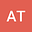POSITIVE PERIODIC SOLUTIONS OF SECOND ORDER DIFFERENTIAL EQUATIONS WITH NONLINEAR NEUTRAL TERM
•• A.K. Tripathy
A.K. Tripathy
Sambalpur University

Corresponding Author:[email protected]

Author Profile## Abstract

\begin{abstract} In this work, we discuss the existence of positive periodic solutions of a class of second order nonlinear neutral delay differential equations of the form \\ \begin{align*} & [u(t)-p(t)f(u(t-\alpha))-q(t)g(u(t-\beta))]’‘= -\sigma(t) u(t)+ h(t, u(t-\alpha), u(t-\beta))\\ & [u(t)-p(t)f(u(t-\alpha))-q(t)g(u(t-\beta))]”= \sigma(t) u(t)- h(t, u(t-\alpha), u(t-\beta)) \end{align*} by using Krasnoselskii’s fixed point theorem. \\ {\bf{Mathematics subject classification (2010)}}: 34K13, 34A34\\ {\bf{Keywords}:} Periodic solution, neutral differential equation, delay, nonlinear.\\ \\ \end{abstract}